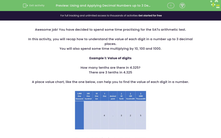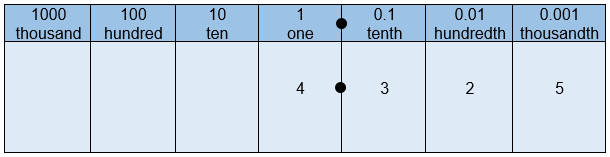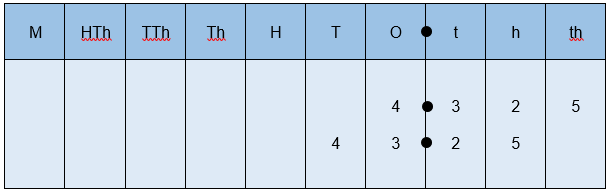# Using and Applying Decimal Numbers up to 3 Decimal Places

In this worksheet, students will recap the place value of numbers with up to 3 decimal places. This worksheet will also rehearse how to multiply decimal numbers by 10, 100 and 1000. These place value skills need to be cemented for the SATs Arithmetic paper.This content is premium and exclusive to EdPlace subscribers.Key stage:  KS 2

Curriculum topic:   Exam-style Questions: SATs Maths

Curriculum subtopic:   Exam-Style Questions: Accurate Decimal Calculations

Difficulty level:#### Worksheet Overview

Awesome job! You have decided to spend some time practising for the SATs arithmetic test.

In this activity, you will recap how to understand the value of each digit in a number up to 3 decimal places.

You will also spend some time multiplying by 10, 100 and 1000.

Example 1: Value of digits

How many tenths are there in 4.325?

There are 3 tenths in 4.325

A place value chart, like the one below, can help you to find the value of each digit in a number.Example 2: Multiplying by 10, 100 and 1000

When multiplying any number by 10, 100 and 1000, the digits move to the left and zero is used as a place holder.

4.325 x 10 = 43.25....All of the digits have moved one place to the left.

4.325 x 100 = 432.5....All of the digits have moved two places to the left.

4.325 x 1000 = 4325....All of the digits have moved three places to the left.

The decimal point does not move.

When multiplying by 10, 100 and 1000, you can again use a place value chart to ensure you put each digit in the correct place!

Below you can see how each digit moves when 4.325 is multiplied by 10.Now, over to you to try some of the practice questions...good luck!

### What is EdPlace?

We're your National Curriculum aligned online education content provider helping each child succeed in English, maths and science from year 1 to GCSE. With an EdPlace account you’ll be able to track and measure progress, helping each child achieve their best. We build confidence and attainment by personalising each child’s learning at a level that suits them.

Get started